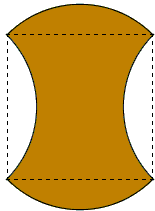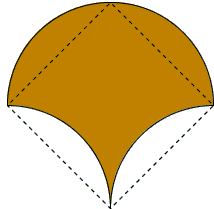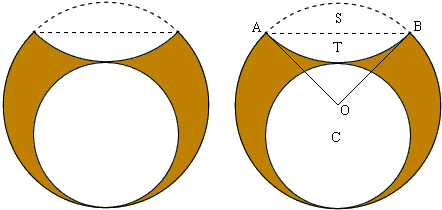# Quadrature: A Child's Play

To affect a quadrature of a plane shape is to construct a square with the same area. For this reason, the task is often referred to as squaring the shape. One of the most famous problems of antiquity is the problem of squaring the circle. According to the Greek tradition, the construction must be carried out with ruler and compass, which, by now, is known to be impossible.

The problem is solvable for every polygon, rectangle in particular. These are known as rectilinear shapes due to the fact that their boundaries consist of straight line segments. Circle and circular arcs come next in complexity. Lunes, i.e. concave shapes bounded by two circular arcs, have been tackled by such luminaries as Hippocrates of Chios, a pupil of Pythagoras, in the fifth century B.C., Alhazen (965-1040), and Leonardo da Vinci (1452-1519). From the sixth century A.D. commentator Simplicius' rendition of Eudemus' History of Geometry (Eudemus of Rhodes (4th century B.C.)) we learn of the variety of lunes squared by Hippocrates. Either one or the other is responsible for the false claim that Hippocrates was able to square all possible lunes.

Some quadratures are pretty obvious even to young children, especially those that are equidecomposable with a square. The simplest examples are served by analogy with the solution to the Twelve Matchsticks Area Puzzles, the executioner's ax belowand a shape that resembles an open parachute or, perhaps, a dandellion of an unusual color.Another example [Martin, p. 27], known as Leonardo's Claws, requires more ingenuity and algebraic dexterity.(Another of Leonardo's mathematical exploits can be found elsewhere.)

## References

1. W. Dunham, Journey through Genius, Penguin Books, 1991
2. J. Fauvel, J. Gray (eds), The History of Mathematics. A Reader, The Open University, 1987
3. G. E. Martin, Geometric Constructions, Springer, 1998### Quadrature: A Child's PlayLet R be the radius of the big circle, from which we cut two equal circular segments (S and T) with central angles 90o and a circle C which touches the big circle and the segment T.The altitude in the isosceles ΔAOB equals R/2. The height of either S or T is then

R - R/2,

and that of the lens they form together is double that:

2R - R2.

Subtracting this from the diameter 2R of the big circle gives the diameter of the small circle: R2. The area of the small circle then is πR²/2. The area of segments S and T is the difference of the area of the circular sector (πR²/4) and the triangle (R²/2). As the result, the area of the claws is:

πR² - 2(πR²/4 - R²/2) - πR²/2 = R².